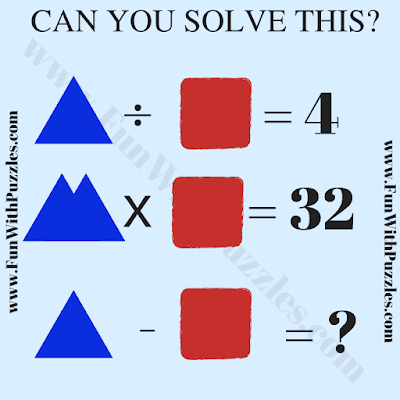This is an interesting math brain teaser which will test your mathematical skills. In this math picture puzzle, there are algebraic equations which you need to solve to get the values of the Triangle and Square. Once you are able to find these values, solve the last equation. Let's see how much time you will take to solve these maths equations?Can you solve this Math Picture Puzzle?

The answer to this "Math Brain Teaser", can be viewed by clicking on the answer button.